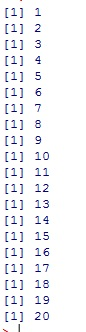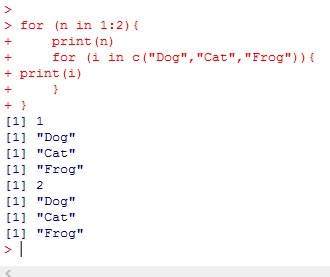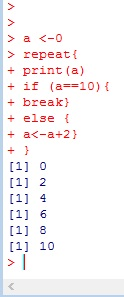# R: Loops – For, While, Repeat

Loops are how we get computers to repeat tasks. In R, there are three standard loops: For, While, and Repeat

## For

The For loop is a simple loop that iterates through a set of elements. With each iteration (running of the loop) the action found inside the loop is repeated.

In the loop I created below, 1:20 means 1 through 20. i in 1:20 mean count 1 through 20, assigning the current value of the count to i for the iteration of the loop.Here are the resultsYou can use a vector to iterate through. You can even use strings.### Nested Loop

You can even nest your loops (running a loop inside another loop)

My main loop counts to 2. Each time it runs it prints its count and then it runs a second loop that steps through a vector containing 3 strings. It prints out each string in order, then returns to the top of the main loop and does it one more time.## While loop

While loops work off of conditional logic. The general concept is while some condition is True, the loop will iterate. Once the condition is False, the loop terminates.

Below is state, while c is less than 10, iterate through the loop. Inside the loop I created a counter that adds 2 to the value of c each time. The end result is, I have created a listing of even numbers from 0 to 8## Repeat Loop

Repeat loops repeat themselves (shocking!! I know!) until they are terminated using the break command.

Note the break command can be used in For and While loops if needed.## The Code

```# loop prints numbers 1 - 20
for (i in 1:20){
print (i)
}

# loop prints elements in vector
for (i in c("Dog","Cat","Frog")){
print(i)
}

#nested loop
for (n in 1:2){
print(n)
for (i in c("Dog","Cat","Frog")){
print(i)
}
}

#while loop
c <- 0
while (c<10){
print(c)
c <- c+2
}

#repeat loop
a <-0
repeat{
print(a)
if (a==10){
break}
else {
a<-a+2}
}```